Function Repository Resource:

# HexagonalTorusGraph

Get a skew graph on a torus with a given number of hexagonal cells

Contributed by: Ed Pegg Jr
 ResourceFunction["HexagonalTorusGraph"][hexagons,{a,b}] makes an (a,b)-skew torus graph with a given number of hexagons.

## Details and Options

Some sextic circulant graphs have toroidal embeddings. Most of the time, the toroidal dual of circulant graph Cn(a,b,a+b) is the (a,b)-skew hexagonal torus graph.
Duals of circulant graphs C9(1,2,4), C12(1,2,5), C18(1,2,8) and C20(1,2,9) cannot be made with this method. The Shrikhande graph dual is another exception.

## Examples

### Basic Examples (2)

There is a unique hexagonal torus graph with eight cells:

 In:=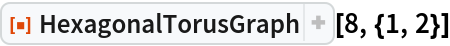Out=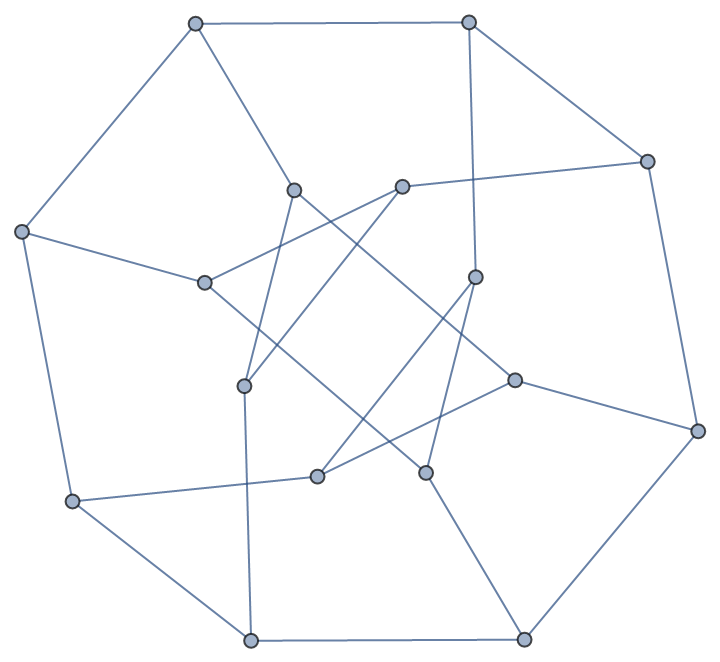A 30-hexagon toroidal graph in 3D:

 In:=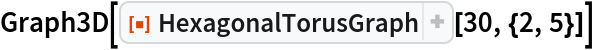Out=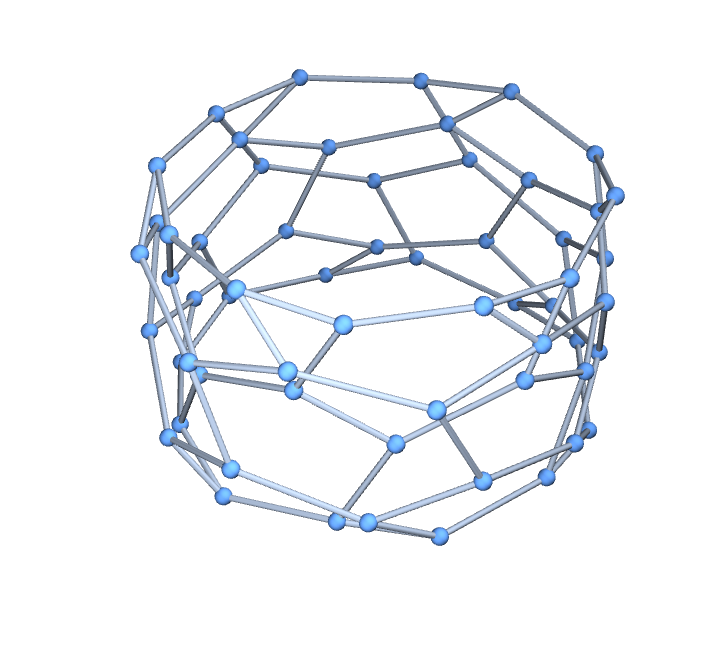### Scope (1)

When a hexagonal torus graph of this form exists, the toroidal dual is the circulant graph (a, b, a+b):

 In:=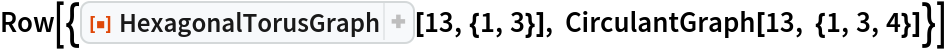Out=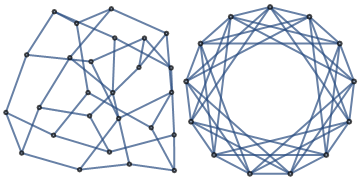### Possible Issues (3)

Some skew values are invalid:

 In:=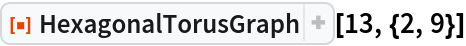Out=The torus graphs corresponding to the toroidal duals of the following sextic toroidal graphs up to 20 vertices are out of scope for this function:

 In:=The number of distinct torus graphs with 7 to 100 hexagons is given by the following code (see A129033):

 In:=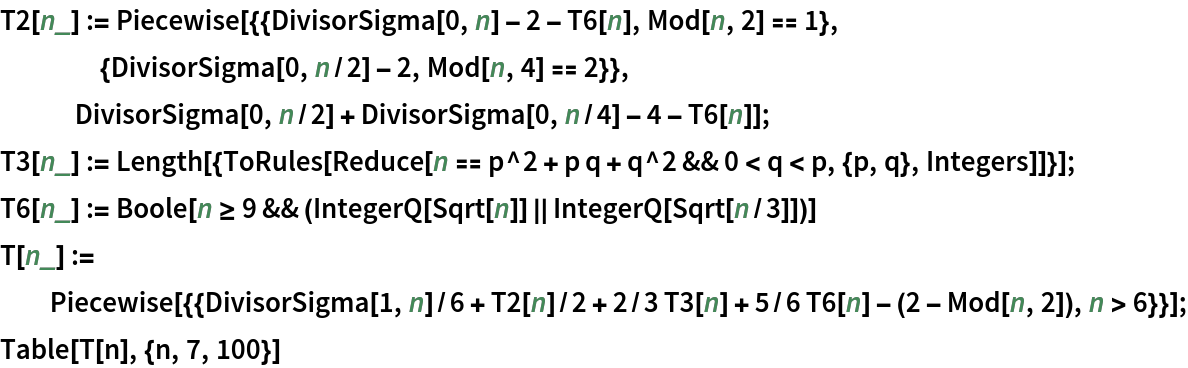Out=### Neat Examples (1)

The 33 distinct hexagonal torus graphs up to 20 hexagons that can be made with this method:

 In:=Out=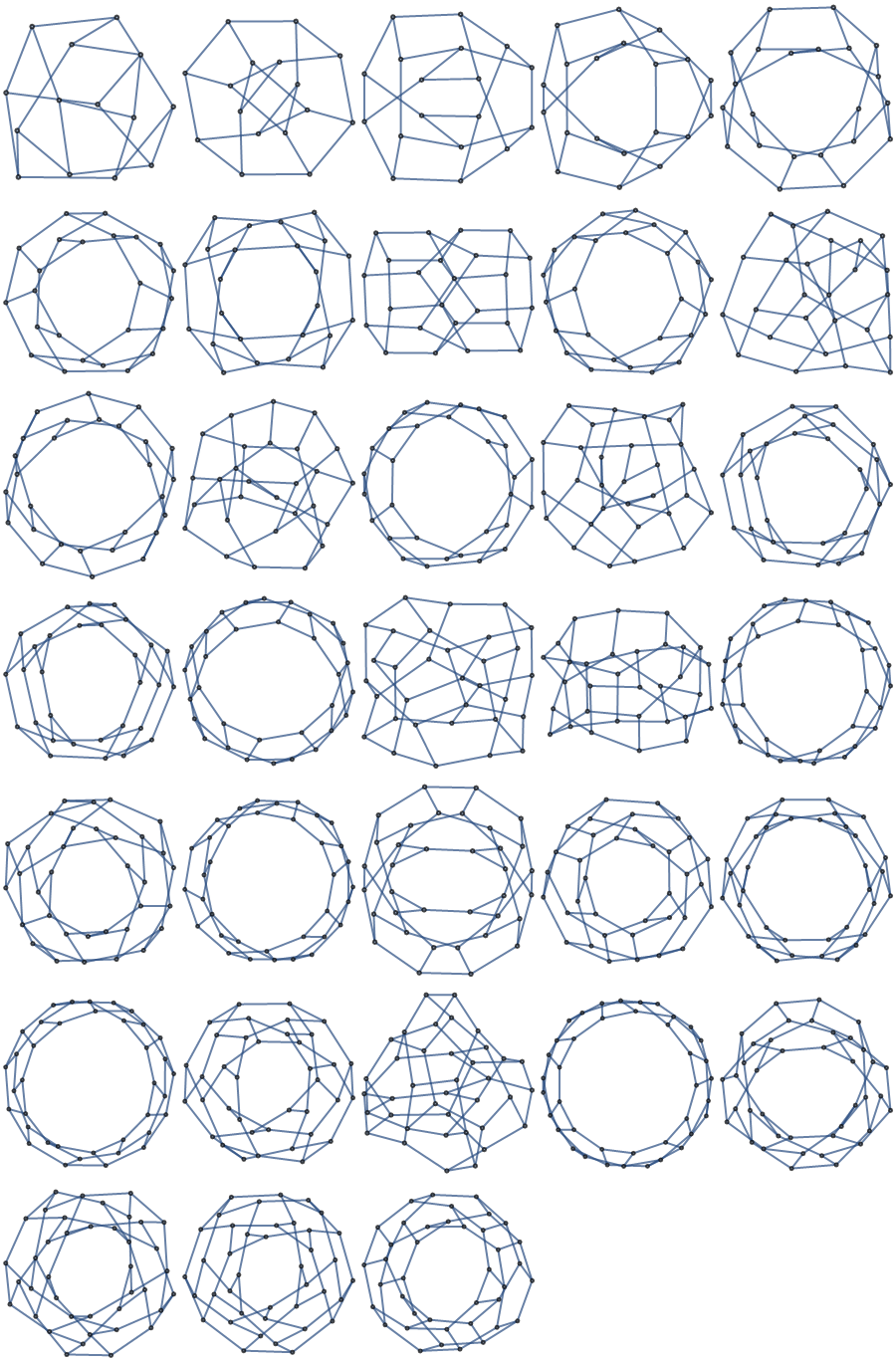## Version History

• 2.0.0 – 28 February 2020
• 1.0.0 – 05 February 2020